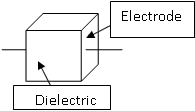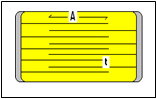# Capacitor Basics

A Capacitor is a basic storage device used to store electrical charges and release it as it is required by the circuit. In a simple form, it is made of two conductive plates (Electrodes) and an insulating medium (Dielectric) which separates the electrodes.

Figure 1. Basic Single Layer
CapacitorAs the voltage applies between the opposite electrode plates of a capacitor, an electrical field takes place between the plates and a charge starts accumulating on the surface of the electrodes.

The charges (Q) on the capacitor plates depend on the voltage (V)and the capacitance value (C) and is as follows:

Q=C*V

Capacitors charge exponentially versus time and the charging current follows the following formula:

I=dQ/dt=C*dV/dt

The unit of capacitance measurement is in Farad (F). Due to the large value of Farad, capacitance is typically mentioned as a fraction of Farad as follows:

There is a wide variety of dielectrics in the capacitor industry. Each dielectric is characterized by a number of specifications, including its ability to store energy (dielectric constant K), dielectric strength, insulation resistance, temperature coefficient (TC), high Frequency performance and more.

A Multilayer capacitor, as its name implies, is constructed in multiple layers.  This allows the manufacturer to achieve higher capacitance values. (Fig 2)

Figure 2: Multilayer CapacitorThe capacitance of a multilayer capacitor is calculated as Follows:

C=K*A*n/4.44*t

C: Capacitance in uF K: Dielectric Constant
A: Active area (in2) n: Number of layers
t: Active dielectric thickness (in2)

As one can see, a higher capacitance value can be achieved by increasing the number of layers (n), decreasing the dielectric thickness (t), larger size or using higher dielectric constant
(K) materials.
Typical ceramic capacitors are made with Barium Titanate (BaTio3) material. The dielectric constant of some of the common dielectrics used in the industry is as follows:

 Vacuum 1 Air 1.004 Mylar 3 Paper 5
 Mica 6.0 Glass 4.0-18.0 Barium Titanate 1500-2000 Other formulated ceramics 8000-20000